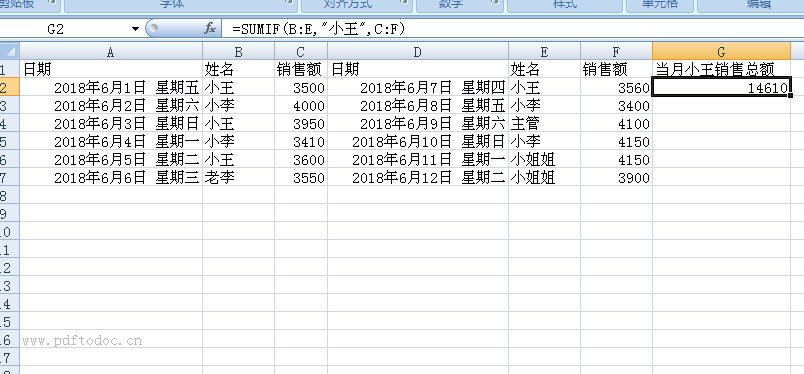# Excel中SUMIF函数的14种用法你会了吗？

=SUMIF(条件区域,指定的条件,需要求和的区域)如果省略求和区域，会将条件区域作为求和区域。

SUMIF函数真的这么简单吗？今天咱们就一起揭开SUMIF函数清纯的面纱，领略一下她的盖世武功。

1、小王的销售额

=SUMIF(B:B,"小王",C:C)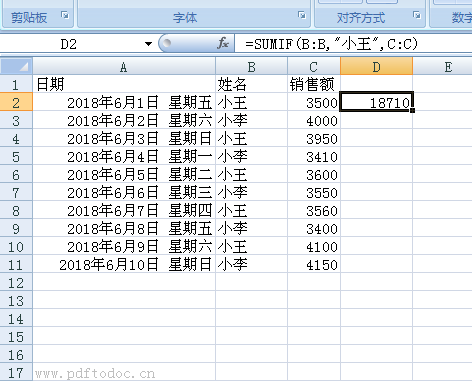2、除了小王之外的销售额

=SUMIF(B:B,"<>小王",C:C)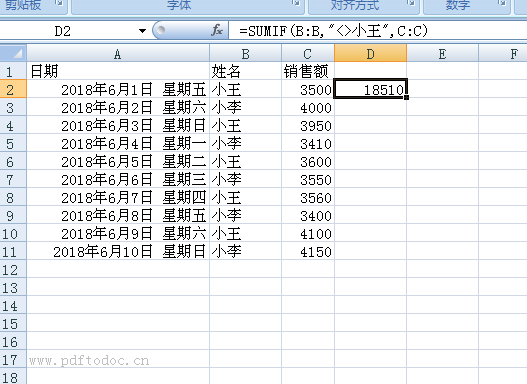3、大于3500的销售额
=SUMIF(C:C,">3500")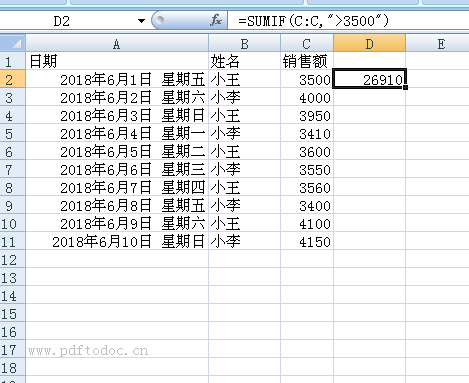4、低于平均值的销售总额
=SUMIF(C:C,"<"&AVERAGE(C:C))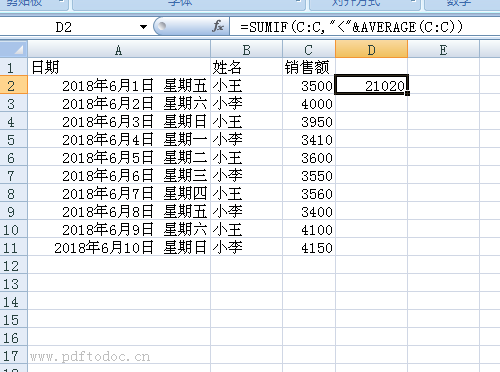5、等于单元格中姓名的销售额
=SUMIF(B:B,E1,C:C)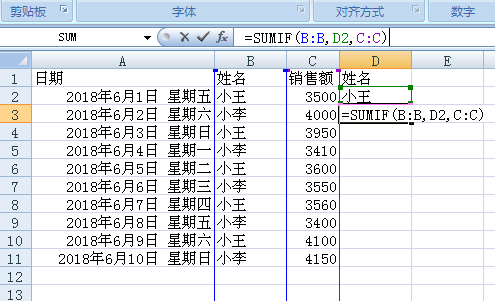6、姓名为三个字的销售额
=SUMIF(B:B,"???",C:C)8、姓名中包含“小”销售额
=SUMIF(B:B,"**",C:C)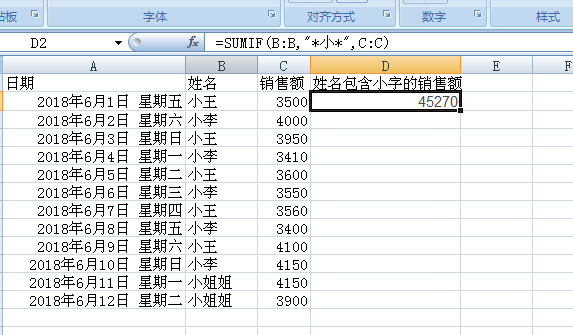9、姓名以“小”开头的销售额
=SUMIF(B:B,"小*",C:C)

10、返回当前日期的销售额
=SUMIF(A:A,TODAY(),C:C)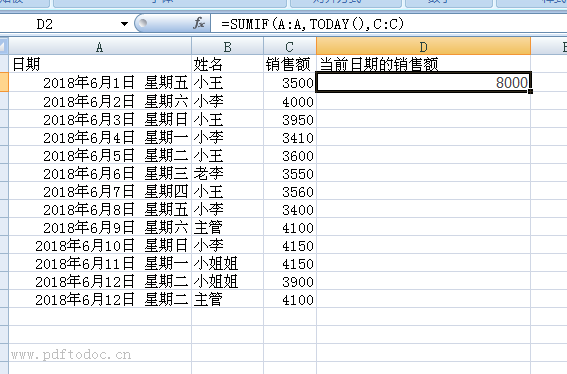11、计算带有错误值的销售额
=SUMIF(C:C,"<9e307")

12、返回小王和小李的销售总额
=SUM(SUMIF(B:B,{"小王","小李"},C:C))

13、每个人最后一次考核分数的平均分
=SUMIF(B3:F10,"",B2:F9)/5

14、返回小王的销售总额
=SUMIF(B:E,"小王",C:F)